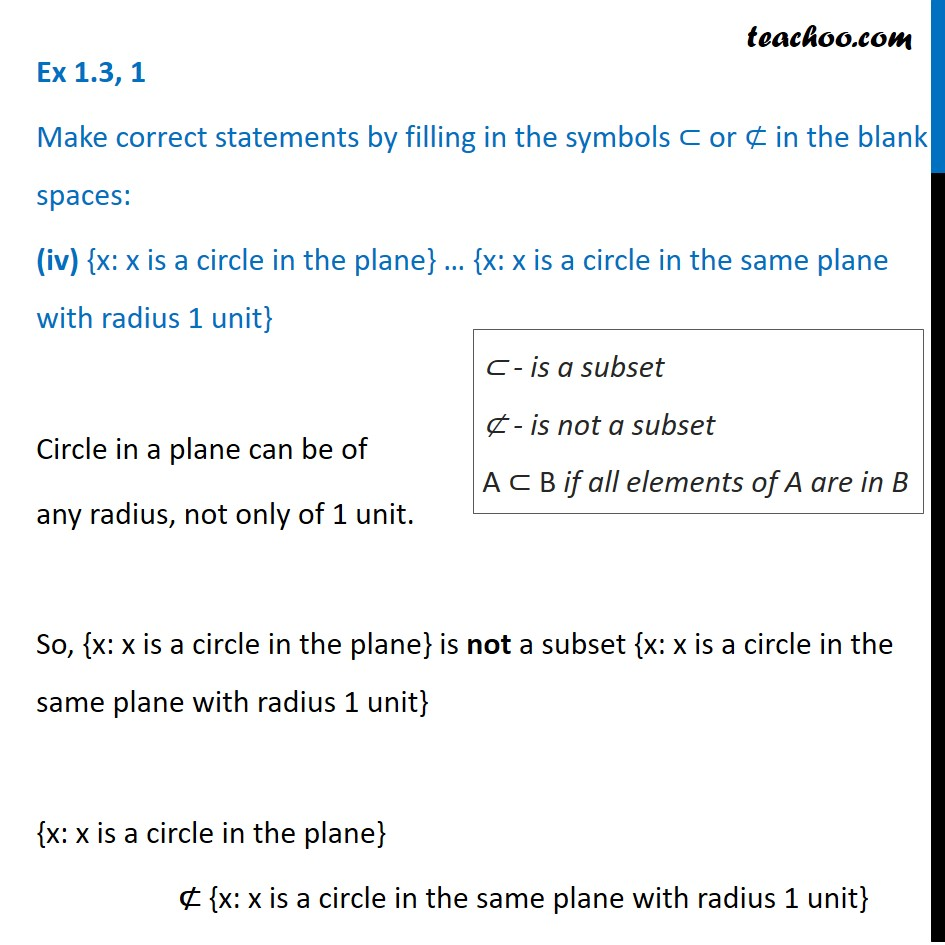Ex 1.3

Chapter 1 Class 11 Sets
Serial order wiseLearn in your speed, with individual attention - Teachoo Maths 1-on-1 Class

### Transcript

Ex 1.3, 1 Make correct statements by filling in the symbols ⊂ or ⊄ in the blank spaces: (iv) {x: x is a circle in the plane} … {x: x is a circle in the same plane with radius 1 unit} Circle in a plane can be of any radius, not only of 1 unit. So, {x: x is a circle in the plane} is not a subset {x: x is a circle in the same plane with radius 1 unit} {x: x is a circle in the plane} ⊄ {x: x is a circle in the same plane with radius 1 unit} ⊂ - is a subset ⊄ - is not a subset A ⊂ B if all elements of A are in B